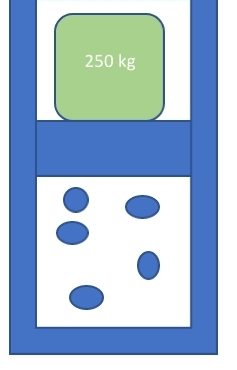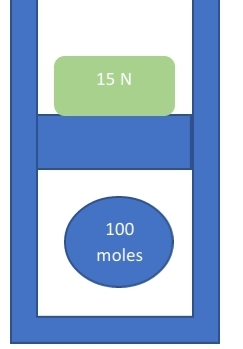# How to Find the Direction of Acceleration of a Piston

## How to Find the Direction of the Acceleration of a Piston

Step 1: Read the problem, picture the scene, and the draw an image depicting what is happening within the problem. This will provide a visual that you can reference back to if needed.

Step 2: Write down all the formulas that apply to the problem. For this particular problem, we will be using the following formulas as seem below:

W=mg , where m represents mass and g represents gravity. This will be used to calculate the weight of object

PV = nRT, where the variables are as follows: P is pressure, V is volume, n is the number of moles, R is the ideal gas constant which is 0.08206 L atm {eq}mol^{-1} {/eq} {eq}K^{-1} {/eq} and T is temperature in Kelvins. This will be used to calculate any of those variables if all are not provided.

W- PA, where W represents weight, P represents pressure, and A represents the cross sectional area of the piston. This will be used to calculate in which direction the piston will accelerate. If the difference is positive, the piston will accelerate downward. If the difference is negative, the piston will accelerate upward.

Step 3: Use to formulas and the information provided to solve the problem.

## What is a Piston?

A piston is a disk within a cylinder that can move upward or downward.

## What is Newton's Second Law?

This law states that the sum of all forces acting on an object are equal to the force times the acceleration of the object as seen below:

{eq}F=ma {/eq}

## What is the Ideal Gas Law?

The ideal gas law states that there is a relationship between pressure, volume, and temperature

The following examples will illustrate how to determine the direction of acceleration of a Piston

## Example 1

A mass of 250 kg sits on top of a piston. The piston has a volume of 4.00 L and a cross sectional area of 0.07 {eq}m^{2} {/eq}. Inside the piston are 5 moles of an ideal gas at a temperature of 200 K. Determine the Direction in which the piston will accelerate.When an object is placed on the piston, two forces will act on the piston: the weight W of the object, which is calculated from mass times gravity. We know this will be a downward force because gravity is a downward force. We also know that the force the gas exerts on the piston, which will downward as well. This force will be the result of PA, which equals which equals to the pressure of the gas and A is the cross sectional area of the piston. Therefore using the formulas listed above in Step 2, we get the following:

Using W=mg, we can determine the weight of the mass

(250)(9.8) = 2450 N

Using PV = nRT to determine Pressure, we get the following

,

(P) (4.00 L) = (5 mol) (0.08206 L atm {eq}mol^{-1} {/eq} {eq}K^{-1} {/eq} )(200 K)

P = 20.515 atm

Using Newton's Second law, we can determine that mg- PA= 0. From this, we can plug in the values we have and if the difference is positive, we know the piston will accelerate downward and if the difference is negative, it will accelerate upwards. Using the formula, we get the following:

2450 - (20.515)(0.07)

2450 - 1.44 = 2448.56

Since this difference is positive, the piston will move downward

## Example 2

A mass with a weight of 15 N is placed on top of a piston. The piston, with a volume of 3.00 L, contains 100 moles of an ideal gas, its temperature is 600 K, and it has a cross sectional are of 0.05 {eq}m^{2} {/eq}. Determine which direction the piston will accelerate.As seen in the above example, using the Ideal Gas Law we can solve for pressure. Once we do this, we can then apply Newton's Second Law and determine if the difference is positive or negative

Using the Ideal Gas Law, we get

P (3) = (100 mol) (0.08206 L atm {eq}mol^{-1} {/eq} {eq}K^{-1} {/eq} )(600 K)

P= 4923.6atm

Using Newton's Second Law, we get

W -PA

15 - (4923.6)( 0.05)

15 - 246.18 = -231.18

Since the difference is negative, we know that the piston will accelerate upward.